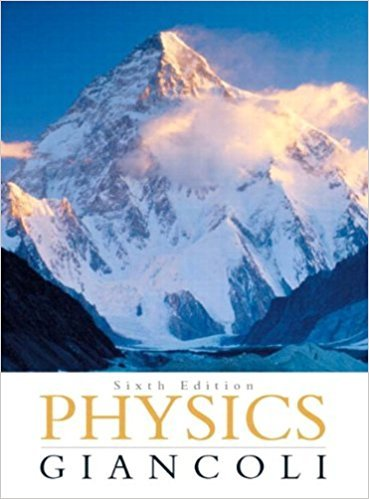×
×

# (II) A particle at t\= -2.0s is at .V| = 3.4cm and at t2 =ISBN: 9780321569837 99

## Solution for problem 6 Chapter 2

Physics: Principles with Applications | 6th Edition

• Textbook Solutions
• 2901 Step-by-step solutions solved by professors and subject experts
• Get 24/7 help from StudySoup virtual teaching assistantsPhysics: Principles with Applications | 6th Edition

4 5 1 274 Reviews
25
1
Problem 6

(II) A particle at t\= -2.0s is at .V| = 3.4cm and at t2 = 4.5 s is at x2 = 8.5 cm. What is its average velocity? Can you calculate its average speed from these data?

Step-by-Step Solution:
Step 1 of 3
Step 2 of 3

Step 3 of 3

##### ISBN: 9780321569837

Unlock Textbook Solution